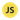# Pass By Value And Pass By Reference In JavaScript

//Edit✏️

Until recently, I always think that variables in Javascript are passed by value. BAM!

In this post, we will look into pass by value and pass by reference in Javascript.

Let’s see what is pass by value and pass by reference before looking into its javascript context.

## Pass by Value:

In Pass by Value, Function is called by directly passing the value of the variable as the argument.

Changing the argument inside the function does NOT affect the variable passed from outside the function.

Javascript always pass by value so changing the value of the variable never changes the underlying primitive (String or number).

``````function callByValue(varOne, varTwo) {
console.log("Inside Call by Value Method");
varOne = 100;
varTwo = 200;
console.log("varOne =" + varOne +"varTwo =" +varTwo);
}

let varOne = 10;
let varTwo = 20;
console.log("Before Call by Value Method");
console.log("varOne =" + varOne +"varTwo =" +varTwo);
callByValue(varOne, varTwo)
console.log("After Call by Value Method");
console.log("varOne =" + varOne +"varTwo =" +varTwo);``````

#### The output will be:

``````Before Call by Value Method
varOne =10 varTwo =20
Inside Call by Value Method
varOne =100 varTwo =200
After Call by Value Method
varOne =10 varTwo =20``````

However, when a variable refers to an object which includes array, the value is the reference to the object.

## Pass by Reference:

In Pass by Reference, function is called by directly passing the reference/address of the variable as the argument.

Changing the argument inside the function affect the variable passed from outside the function.

In Javascript, objects and arrays are passed by reference.

``````function callByReference(varObj) {
console.log("Inside Call by Reference Method");
varObj.a = 100;
console.log(varObj);
}

let varObj = {a:1};
console.log("Before Call by Reference Method");
console.log(varObj);
callByReference(varObj)
console.log("After Call by Reference Method");
console.log(varObj);``````

#### The output will be:

``````Before Call by Reference Method
{a: 1}
Inside Call by Reference Method
{a: 100}
After Call by Reference Method
{a: 100}``````

So if we are passing object or array as an argument to the method, then there is a possibility that value of the object can change.

## Summary

The most important thing to remember is:

• In Javascript, objects and arrays are passed by reference.
• Other primitive values are passed by value.

Hope you find this information useful. Good bye!